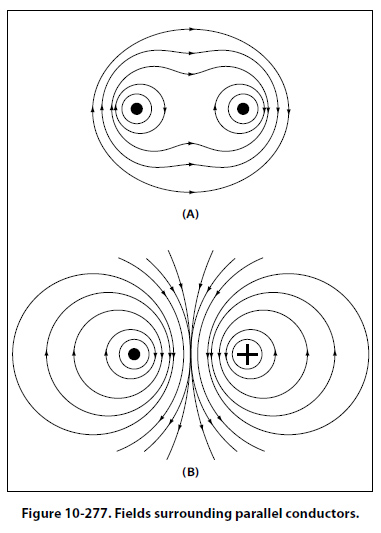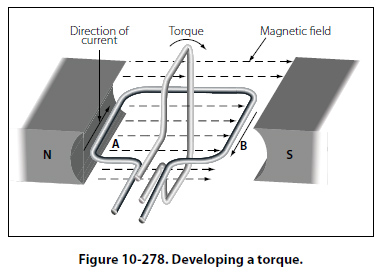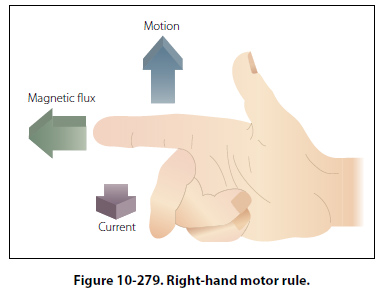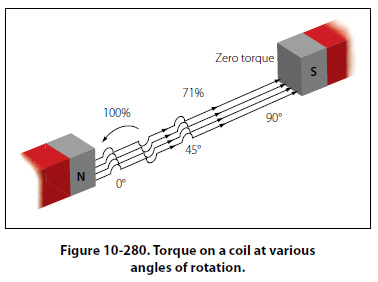Force between Parallel Conductors Two wires carrying current in the vicinity of one another exert a force on each other because of their magnetic fields. An end view of two conductors is shown in Figure 10-277. In A, electron flow in both conductors is toward the reader, and the magnetic fields are clockwise around the conductors. Between the wires, the fields cancel because the directions of the two fields oppose each other. The wires are forced in the direction of the weaker field, toward each other. This force is one of attraction. In B, the electron flow in the two wires is in opposite directions.The magnetic fields are, therefore, clockwise in one and counterclockwise in the other, as shown. The fields reinforce each other between the wires, and the wires are forced in the direction of the weaker field, away from each other. This force is one of repulsion. To summarize: conductors carrying current in the same direction tend to be drawn together; conductors carrying current in opposite directions tend to be repelled from each other. Developing Torque If a coil in which current is flowing is placed in a magnetic field, a force is produced which will cause the coil to rotate. In the coil shown in Figure 10-278, current flows inward on side A and outward on side B. The magnetic field about B is clockwise and that about A, counterclockwise. As previously explained, a force willdevelop which pushes side B downward. At the same time, the field of the magnets and the field about A, in which the current is inward, will add at the bottom and subtract at the top. Therefore, A will move upward. The coil will thus rotate until its plane is perpendicular to the magnetic lines between the north and south poles of the magnet, as indicated in Figure 10-278 by the white coil at right angles to the black coil. The tendency of a force to produce rotation is called torque. When the steering wheel of a car is turned, torque is applied. The engine of an airplane gives torque to the propeller. Torque is developed also by the reacting magnetic fields about the current carrying coil just described. This is the torque, which turns the coil. The right-hand motor rule can be used to determine the direction a current carrying wire will move in a magnetic field. As illustrated in Figure 10-279, if the index finger of the right hand is pointed in the direction of the magnetic field and the second finger in the direction of current flow, the thumb will indicate the direction the current carrying wire will move.The amount of torque developed in a coil depends upon several factors: the strength of the magnetic field, the number of turns in the coil, and the position of the coil in the field. Magnets are made of special steel that produces a strong field. Since there is torque acting on each turn, the greater the number of turns on the coil, the greater the torque. In a coil carrying a steady current located in a uniform magnetic field, the torque will vary at successive positions of rotation, as shown in Figure 10-280. When the plane of the coil is parallel to the lines of force, the torque is zero. When its plane cuts the lines of force at right angles, the torque is 100 percent. At intermediate positions, the torque ranges between zero and 100 percent.©AvStop Online Magazine                                                                                                                                                      Contact Us              Return To Books